Question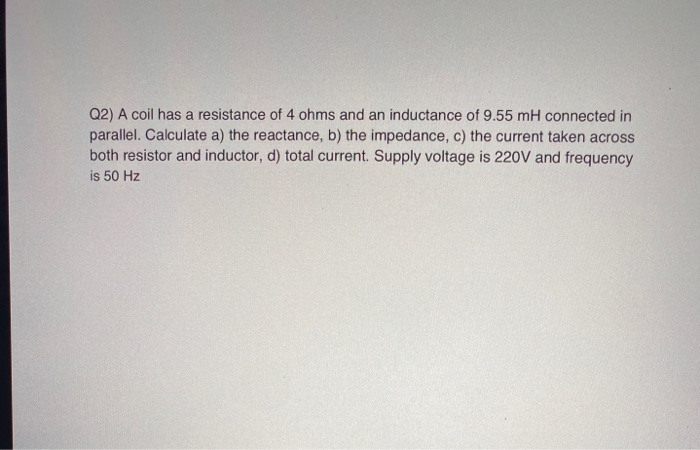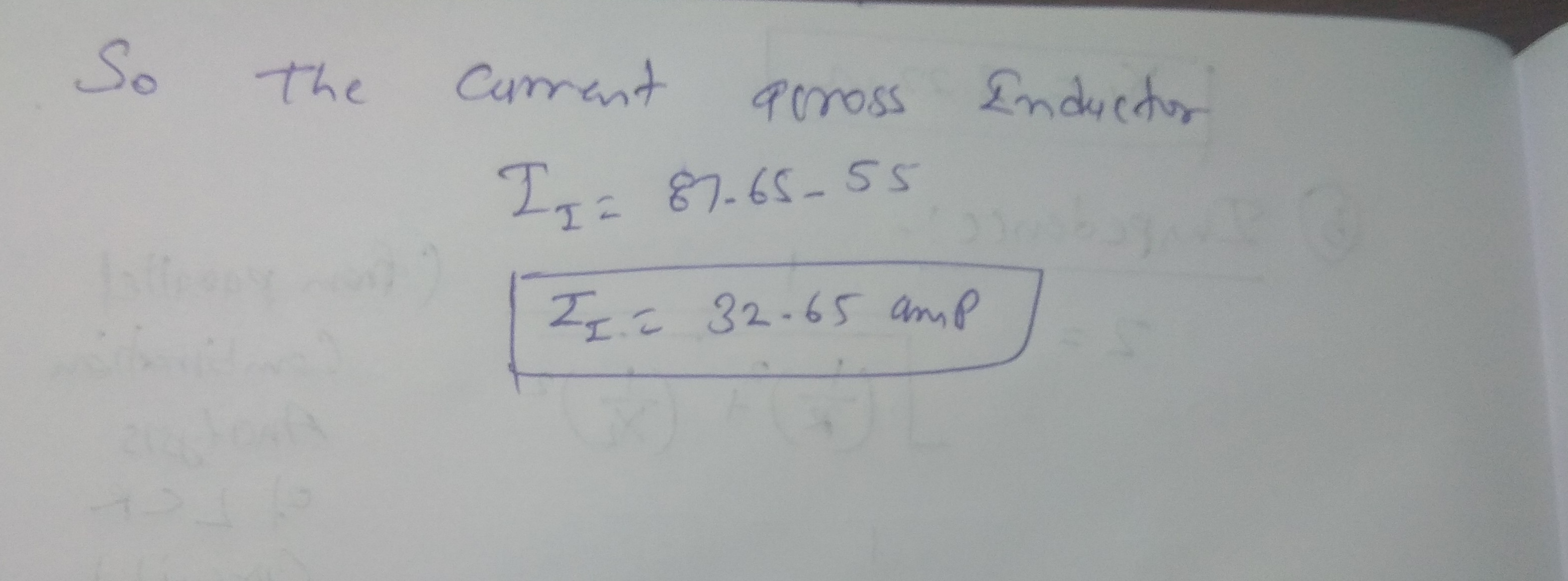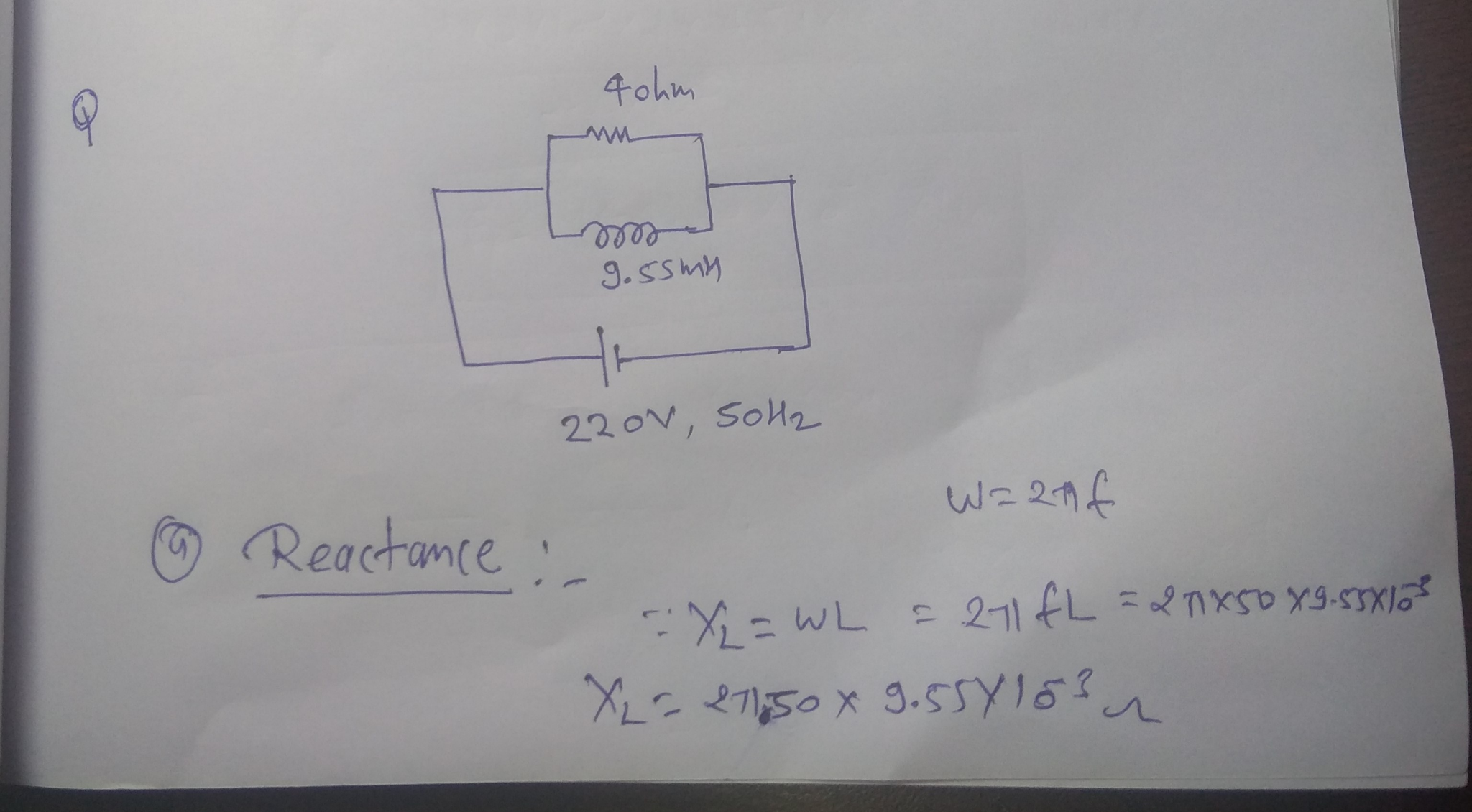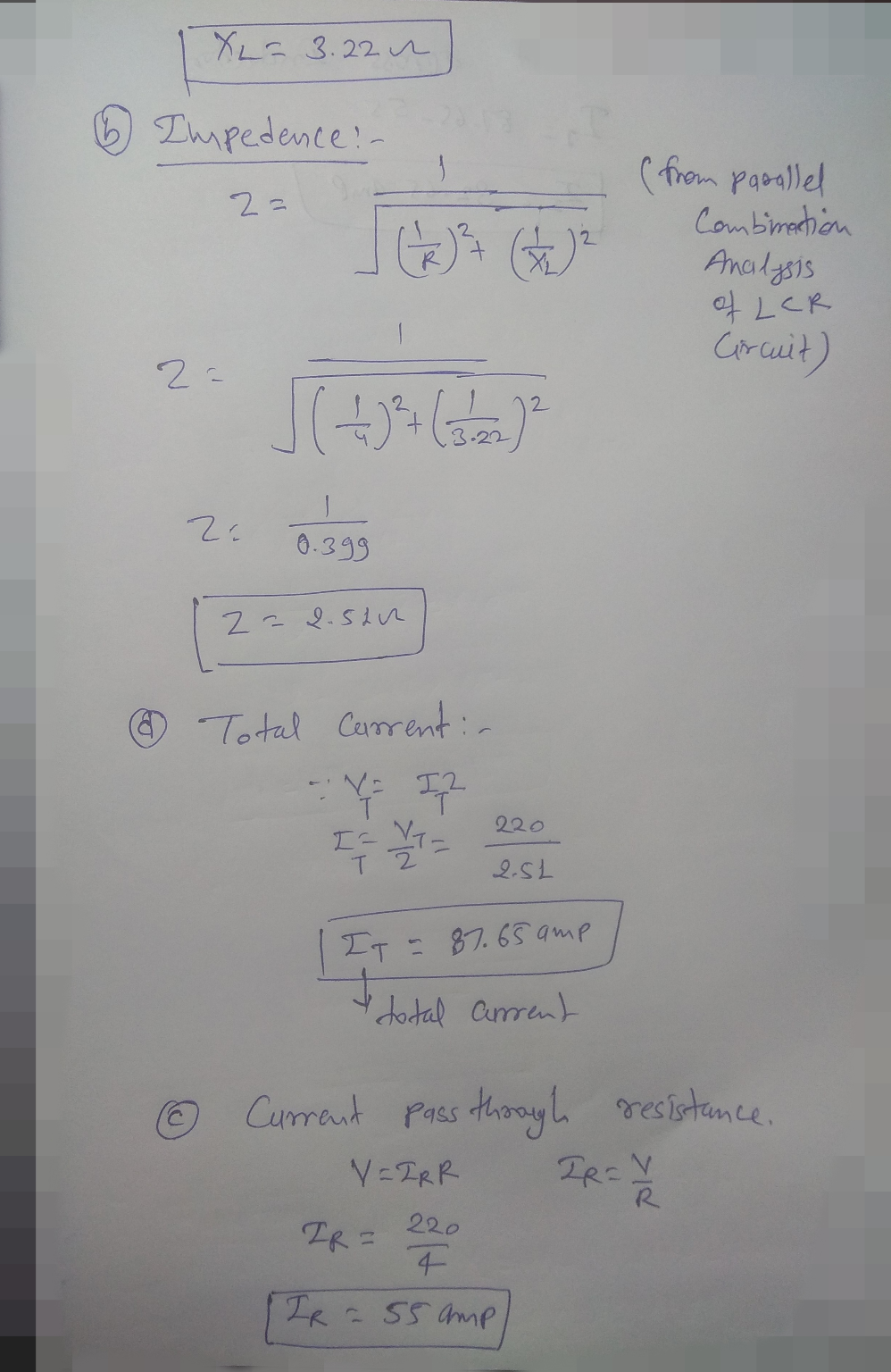#### Earn Coins

Coins can be redeemed for fabulous gifts.

Similar Homework Help Questions
• ### An ideal inductor of inductance 7.7 mH is connected in parallel with a resistor of resistance...

An ideal inductor of inductance 7.7 mH is connected in parallel with a resistor of resistance 141 Ω. This parallel combination is then connected in series with a second ideal inductor of inductance 4.5 mH. The sinusoidal voltage source for the circuit is 102 V, 71.6 Hz. Sketch the circuit and find the magnitude of the current, I, flowing through the resistor.

• ### An AC circuit has a resistance of 500 ohms, a voltage of 200 V, an inductance...

An AC circuit has a resistance of 500 ohms, a voltage of 200 V, an inductance of 0.4 mHz, a capacitance of 100pF and an angular frequency of 5.00 x 105 rad/s. What is the impedance? What is the current amplitude? What is the voltage amplitude read by a voltmeter across the inductor, the resistor, and the capacitor individually? What is the voltage amplitude read by a voltmeter across the inductor and capacitor together?

• ### a) A coil having resistance of 12 2 and an unknown inductance is connected in series...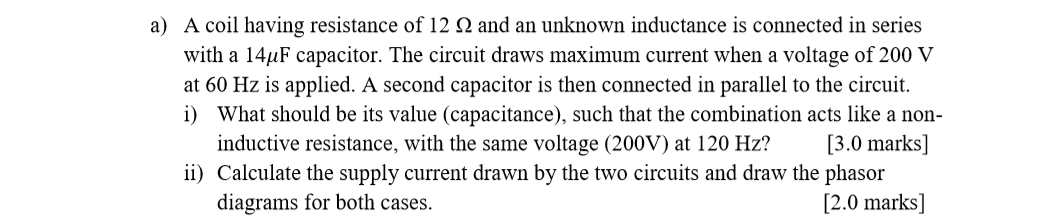a) A coil having resistance of 12 2 and an unknown inductance is connected in series with a 14uF capacitor. The circuit draws maximum current when a voltage of 200 V at 60 Hz is applied. A second capacitor is then connected in parallel to the circuit. i) What should be its value (capacitance), such that the combination acts like a non- inductive resistance, with the same voltage (200V) at 120 Hz? [3.0 marks] ii) Calculate the supply current drawn...

• ### 1. L RC Circuit. An AC circuit has an inductance L -5 mH, a resistance of...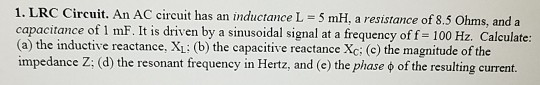1. L RC Circuit. An AC circuit has an inductance L -5 mH, a resistance of 8.5 Ohms, and a capacitance of 1 mF. It is driven by a sinusoidal signal at a frequency of f 100 Hz. Calculate (a) the inductive reactance, XL: (b) the capacitive reactance Xc: (c) the magnitude of the impedance Z: (d) the resonant frequeney in Hertz, and (e) the phase of the resulting current.

• ### RLC resonance 5.2 A A coil with a resistance of 5, and an inductance of 0.03...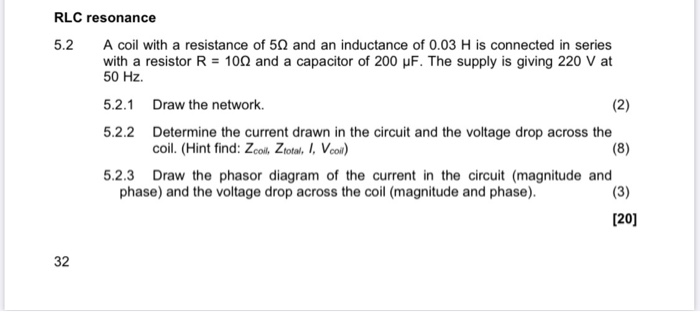RLC resonance 5.2 A A coil with a resistance of 5, and an inductance of 0.03 H is connected in series coil with a with a resistor R = 100 and a capacitor of 200 uF. The supply is giving 220 V at 50 Hz. 5.2.1 Draw the network. (2) 5.2.2 Determine the current drawn in the circuit and the voltage drop across the coil. (Hint find: Zcoil. Ztotal. I, Vcoil) 5.2.3 Draw the phasor diagram of the current in...

• ### An ac circuit with a 60 μF capacitor in series with a coil of resistance 18Ω...

An ac circuit with a 60 μF capacitor in series with a coil of resistance 18Ω and inductance 180mH is connected to a 100V, 100 Hz supply is shown below. Calculate inductive reactance                                                                           capacitive reactance                                                                          circuit impedance and phase angle θ circuit current I                                                                                          phasor voltages VR, VL, VC and VS                                                         resonance circuit frequency                                                                   construct a fully labeled voltage phasor diagram

• ### A coil with an inductance of 8.6 mH is in a circuit with an AC frequency...

A coil with an inductance of 8.6 mH is in a circuit with an AC frequency of 6047 Hz. What is the inductive reactance in ohms? Give your answer to 2 sf.

• ### A coil which has an inductance of 40mH and a resistance of 2 ohms is connected...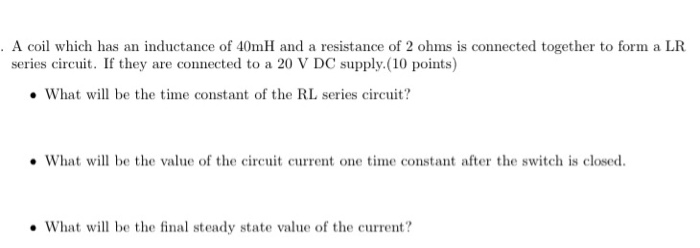A coil which has an inductance of 40mH and a resistance of 2 ohms is connected together to form a LR series circuit. If they are connected to a 20 V DC supply (10 points) • What will be the time constant of the RL series circuit? • What will be the value of the circuit current one time constant after the switch is closed. • What will be the final steady state value of the current?

• ### The rms current in an RL circuit is 0.21 A when it is connected to an...

The rms current in an RL circuit is 0.21 A when it is connected to an ac generator with a frequency of 50 Hz and an rms voltage of 30 V . Part A Given that the inductor has an inductance of 170 mH , what is the resistance of the resistor? Part B Find the rms voltage across the resistor. Part C Find the rms voltage across the inductor.

• ### ogf induced in a coil is 0.72 V when the current in the coil changes to...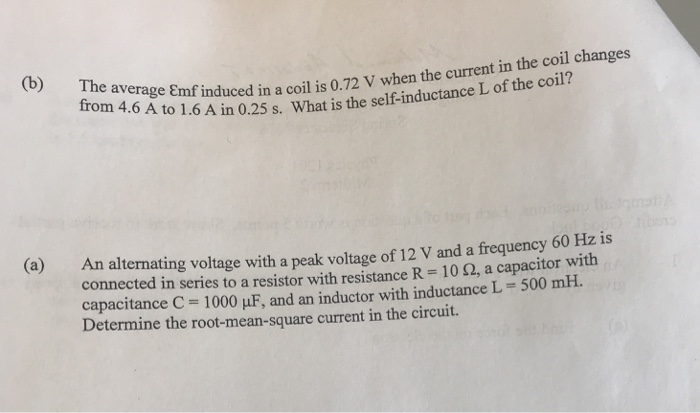ogf induced in a coil is 0.72 V when the current in the coil changes to 1.6 A in 0.25 s. What is the self-inductance L of the coil? An alternating voltage with a peak voltage of 12 V and a frequency 60 Hz is connected in series to a resistor with resistance R : 10 ?, a capacitor with capacitance C-1000 ?F, and an inductor with inductance L 500 mH. Determine the root-mean-square current in the circuit. (a)